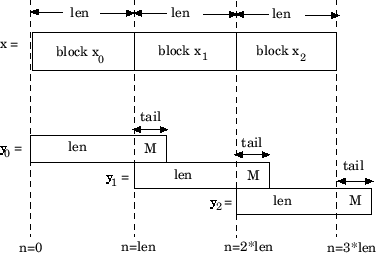Documentation

# dfilt.fftfir

## Syntax

```Hd = dfilt.fftfir(b,len) Hd = dfilt.fftfir(b) Hd = dfilt.fftfir ```

## Description

This object uses the overlap-add method of block FIR filtering, which is very efficient for streaming data.

`Hd = dfilt.fftfir(b,len)` returns a discrete-time, FFT, FIR filter, `Hd`, with numerator coefficients, `b` and block length, `len`. The block length is the number of input points to use for each overlap-add computation.

`Hd = dfilt.fftfir(b)` returns a discrete-time, FFT, FIR filter, `Hd`, with numerator coefficients, `b` and block length, `len=`100.

`Hd = dfilt.fftfir` returns a default, discrete-time, FFT, FIR filter, `Hd`, with the numerator `b=1` and block length, `len=`100. This filter passes the input through to the output unchanged.

### Note

When you use a `dfilt.fftfir` object to filter, the input signal length must be an integer multiple of the object's block length, `len`. The resulting number of FFT points = (filter length + the block length - 1). The filter is most efficient if the number of FFT points is a power of 2.

The `fftfir` uses an overlap-add block processing algorithm, which is represented as follows,where `len` is the block length and `M` is the length of the numerator-1, (`length(b)-1`), which is also the number of states. The output of each convolution is a block that is longer than the input block by a tail of (`length(b)-1`) samples. These tails overlap the next block and are added to it. The states reported by `dfilt.fftfir` are the tails of the final convolution.

## Examples

Create an FFT FIR discrete-time filter with coefficients from a 30th order lowpass equiripple design:

```b = firpm(30,[0 .1 .2 .5]*2,[1 1 0 0]); Hd = dfilt.fftfir(b); % To obtain frequency domain coefficients % used in filtering Coeffs = fftcoeffs(Hd);```